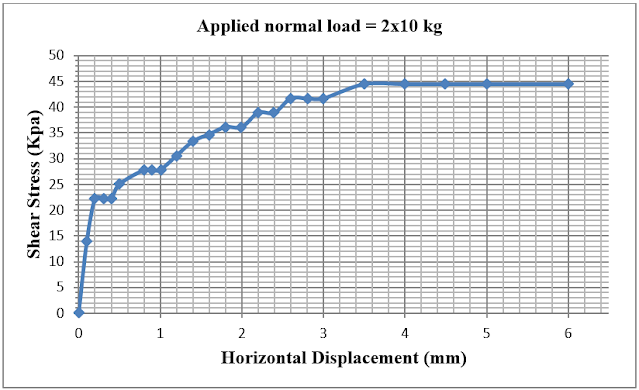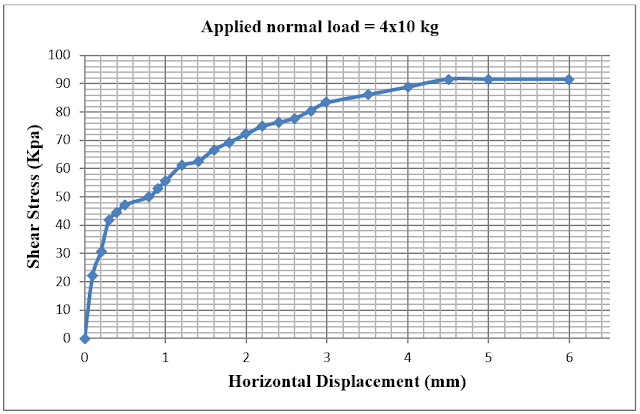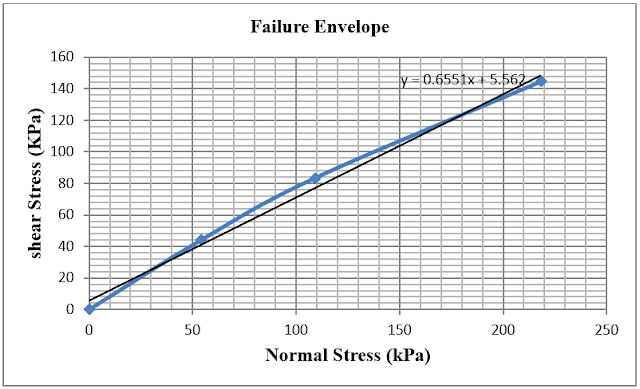### Abstract:

This article represents a method used to determine the shear strength (S) of granular soil. This method is known as the Direct Shear Test on Sand. In this test, a direct shear test machine consisting of a box split into half holds the specimen, and a horizontal load is applied to the specimen. Two dial gauges measure the horizontal and vertical deformations occurring in the specimen, and a proving ring measures the horizontal load applied. Using these values the shear strength of the specimen is then calculated.

### Aim of the experiment:

The aim of this experiment is to determine the value of the shear strength (S) for a granular soil sample.

### Theory:

The shear strength of the soil is defined as the internal resistance the soil offers to resist failure ‎and sliding along any plane inside its structure and thus it is the internal resistance to shear ‎failure. It is important in stability, bearing capacity, and ‎lateral earth pressure analysis.‎ Direct shear test is quick and inexpensive.

Mohr states in the theory of failure, that failure in the soil occur due to a critical combination of shear stress and normal stress. Points having a critical combination of shear and normal stress are located on a line termed the shear envelope.

For almost all types of soil, the failure envelope is a curved line combining all points of critical normal and shear stress values. This original failure envelope is referred to as “Mohr failure envelope” and can be conservatively approximated by a straight line. The straight line relation is referred to as “Coulomb-Mohr failure envelope”. From this straight line the cohesion is determined as the y-intercept and the angle of internal friction is the angle the curve makes with the horizontal axis.

The equations to be used in the calculation process are:‎

The normal stress (σ) and the corresponding shear stress (τ) are calculated using the following ‎equations:

σ = N/A
τ = H/A

Where:
σ = total normal stress on the soil.
τ = shear resistance of the soil at failure point.
H = measured shear force (calculated as shown below)
N = applied normal force (calculated as shown below)
A = cross sectional area of the sand sample.

The applied normal force is calculated using the following equation:

N = Weight of yoke & hanger + (10 * applied load)

The applied shear force is calculated using the following equation:

Moreover, the test carried out is a drained test, so the effective normal stress should be used ‎instead of the total normal stress, thus the equation simplifies to:‎

shear strength = σ' tan Ф

Such that σ' = effective normal stress‎
‎Ф = friction angle of soil

So;
Ф = tan-1 (max shear stress/ normal stress).‎

### Equipments:

Direct shear test machine
Balance sensitive to 0.1 g
Large porcelain evaporating dish
Tamper was used to compact the soil on the box
Spoon

### Procedure:

1. The shear box was filled with sand, the sand was compacted manually and the top surface was leveled with the straight edge.
2. The sand inside the shear box was weighted and weight was recorded.
3. The value of displacement rate was chosen and the gear value was modified.
4. The shear box arrangement was put inside the shear box and the sand in the extension was pushed into the shear box using a piece of wood.
6. The yoke was moved and inserted above the sample in the shear box. The attached wheel was rotated until the attached arm became horizontally located. The yoke was tightened with the attached screw above the sample.
7. The direct shear testing machine was turned on, the reading of the proving ring gauge were recorded at certain time intervals by counting the number of divisions.
8. The previous steps were repeated for two more samples of approximately the same weight. But the load on the vertical yoke arm was increased to 4 kg for the second sample and to 8 kg for the third one.

### Data and calculations:

Table (1): Normal load and stress of the soil sample with its density

First Trial Second Trial Third Trial
Normal Load (Kg) 2*10=20 4*10=40 8*10=80
Normal Stress (KPa) 54.5 109.0 218
Density (gm/cm³) 1.75 1.81 1.81

Table (2): Collected data for the first trial
Horizontal dis. (div) Horizontal dis. (mm) load ring (div) shear force (kN) shear stress (KPa)
0 0 0 0 0
10 0.1 3 0.05 13.89
20 0.2 5 0.08 22.22
30 0.3 5 0.08 22.22
40 0.4 5 0.08 22.22
50 0.5 5.5 0.09 25
60 0.6 6 0.1 27.78
70 0.7 6 0.1 27.78
80 0.8 6 0.1 27.78
90 0.9 6 0.1 27.78
100 1 6 0.1 27.78
120 1.2 6.5 0.11 30.56
140 1.4 7 0.12 33.33
160 1.6 7.5 0.125 34.72
180 1.8 8 0.13 36.11
200 2 8 0.13 36.11
220 2.2 8.5 0.14 38.89
240 2.4 8.5 0.14 38.89
260 2.6 9 0.15 41.67
280 2.8 9 0.15 41.67
300 3 9 0.15 41.67
350 3.5 9.5 0.16 44.44
400 4 9.5 0.16 44.44
450 4.5 9.5 0.16 44.44
500 5 9.5 0.16 44.44
600 6 9.5 0.16 44.44

Table (3): Collected data for the second trial
Horizontal dis. (div) Horizontal dis. (mm) load ring (div) shear force (kN) shear stress (KPa)
0 0 0 0 0.00
10 0.1 5 0.08 22.22
20 0.2 7.5 0.11 30.56
30 0.3 9 0.15 41.67
40 0.4 9.5 0.16 44.44
50 0.5 10 0.17 47.22
60 0.6 10 0.17 47.22
70 0.7 11 0.18 50.00
80 0.8 11 0.18 50.00
90 0.9 11.5 0.19 52.78
100 1 12 0.2 55.56
120 1.2 13 0.22 61.11
140 1.4 13.5 0.225 62.50
160 1.6 14.5 0.24 66.67
180 1.8 15 0.25 69.44
200 2 15.5 0.26 72.22
220 2.2 16 0.27 75.00
240 2.4 16.5 0.275 76.39
260 2.6 17 0.28 77.78
280 2.8 17.5 0.29 80.56
300 3 18 0.3 83.33
350 3.5 18.5 0.31 86.11
400 4 19 0.32 88.89
450 4.5 20 0.33 91.67
500 5 20 0.33 91.67
600 6 20 0.33 91.67

Table (4): Collected data for the third trial
Horizontal dis. (div) Horizontal dis. (mm) load ring (div) shear force (kN) shear stress (KPa)
0 0 0 0 0
10 0.1 10 0.17 47.22
20 0.2 13.5 0.225 62.5
30 0.3 15.5 0.26 72.22
40 0.4 17 0.28 77.78
50 0.5 18 0.3 83.33
60 0.6 19 0.32 88.89
70 0.7 19.5 0.325 90.28
80 0.8 20 0.33 91.67
90 0.9 20.5 0.34 94.44
100 1 21 0.35 97.22
120 1.2 21 0.35 97.22
140 1.4 22 0.37 102.78
160 1.6 22.5 0.38 105.56
180 1.8 23.5 0.39 108.33
200 2 25 0.42 116.66
220 2.2 26.5 0.44 122.22
240 2.4 27.5 0.46 127.78
260 2.6 28.5 0.475 131.94
280 2.8 30 0.5 138.88
300 3 31 0.52 144.44
350 3.5 34 0.57 158.33
400 4 36 0.6 166.67
450 4.5 36 0.6 166.67
500 5 36 0.6 166.67
600 6 36 0.6 166.67

Sample calculation for the first trial:
Cross section area of sample (C.S.A) = 6*6 = 36 cm²
Normal Load (kg) = mass on hanger *10.
Normal Load = 2*10 = 20 kg.
Normal Stress (kPa) = (Normal load * 9.81)/(C.S.A) = (20*9.81)/(36*10) = 54.5 KPa
Horizontal displacement (mm) = read divs *.01 = 10*.01 = 0.1 mm

Shear forces (kN): Values had been taken from table (calibration of 3KN load ring) For each divs reading.

Shear stress (kPa) = shear force / C.S.A

Shear stress = 0.05/0.0036 = 13.89 KPa

Density = Weight of specimen / Volume

Density = (270.1-144) / (72) = 1.75 gm/cm3

### Charts and Graphs:Figure (2): Shear stress Vs. Horizontal Displacement for the first trial

From the graph the maximum shear stress =44.44 kPaFigure (3): Shear stress Vs. Horizontal Displacement for the second trial

From the graph the maximum shear stress = 91.67 kPaFigure (4): Shear stress Vs. Horizontal Displacement for the third trial

From the graph the maximum shear stress = 166.67 kPa

Table (5) :Maximum normal and shear stress
Trial No Maximum normal stress (kPa) Maximum shear stress (kPa)
1 54.5 44.44
2 109.0 83.33
3 218.0 144.4Figure (5): Failure envelope

From the graph, the angle of internal friction can be determined:

Ф = tan-1 [(144.4-44.44) / (218-54.5)] = 31.44°

The cohesion of this soil can be determined from the best fit line of this soil which is clear from the graph to equal to C = 5.562 kPa.

### Results and conclosion

Main result:
C = 5.562 kPa.
Ф = 31.44°

The direct shear strength test is a commonly used technique to determine the shear strength parameters, based on applying a shear force by moving one-half of the box relatively.

The collected data for each trail shows clearly the direct relationship between shear strength and effective normal stress, this agrees with shear strength equation

S= σ̒ tanΦ

This is mainly because when the normal forces increase, the number of contact points also increase thus increasing the resistance

Hence, the shear strength is a function of normal load, angle of friction (amount of interlocking among the soil particles) and cohesion.

As graphs (Applied normal load = 2x10 kg, 4x10 kg, 8x10 kg) show, the resisting shear stress increase with shear displacement until a failure shear stress is reached.

As mentioned above, shear strength is a function of angle of friction Φ which can be determined from slope of the straight line plot of shear stress vs. normal stress .The figure is a straight line passing through the origin for the sand with C = 0 ( cohesion= y intercept), But here the used specimen is dense angular – grained specimen, this is shown clearly from friction angle Φ = 31.44° Engineering use:

The direct shear test on sand is of great importance when it comes to the construction of structures depending on the soil’s shearing resistance. The shear strength is used in determining the stability of slopes or cuts, finding the bearing capacity of the soil, and calculating pressures excreted by the soil on a retaining wall. Therefore, performing such inexpensive test on a granular soil prior to design is crucial, and the design cannot be commenced without the value of the shear strength.

### References:

DAS, Principles of Geotechnical engineering, third edition .